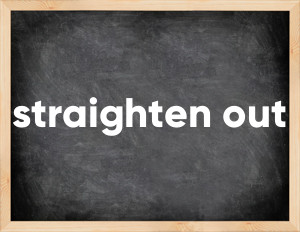# Phrasal verb straighten outEnglish verb straighten out [streɪtn aʊt].
Relate to: phrasal verb.
3 forms of verb straighten out: Infinitive (straighten out), Past Simple - (straightened out), Past Participle - (straightened out).

## Forms of verb straighten out in past tenses

👉 Forms of verb straighten out in future and past simple and past participle.
❓ What is the past tense of straighten out.

## Three forms of verb straighten out

Base Form Past Simple Past Participle
straighten out [streɪtn aʊt]

straightened out [streɪtnd aʊt]

straightened out [streɪtnd aʊt]

## What is 2nd and 3rd form of straighten out?

🎓 Base Form (Infinitive) straighten out in Past Simple, Future Simple, Present Perfect, Past Perfect, Future Perfect?

• First form (V1) - straighten out. (Present simple, Future Simple)
• Second form (V2) - straightened out. (Past simple)
• Third form (V3) - straightened out. (Present perfect, Past perfect)

## What are the past tense and past participle of straighten out?

The past tense of straighten out is: straighten out in past simple is straightened out. and past participle is straightened out.

### What is the past tense of straighten out?

The past tense of straighten out is straightened out.
The past participle of straighten out is straightened out.

### Verb Tenses

Past simple — straighten out in past simple straightened out. (V2)
Future simple — straighten out in future simple straighten out. (will + V1)
Present Perfect — straighten out in present perfect tense straightened out. (have\has + V3)
Past Perfect — straighten out in past perfect tense straightened out. (had + V3)

### straighten out regular or irregular verb?

👉 Is straighten out an irregular verb?? straighten out is .

## Examples of Verb straighten out in Sentences

•   We got a few things to straighten out (Present Simple)
•   Got to straighten out that closet one of these days (Present Simple)
•   So i can straighten out some of her things (Present Simple)
•   He says we can straighten out any of our problems (Present Simple)
•   How can we get this whole thing straightened out? (Present Simple)
•   I can straighten this out (Present Simple)
•   If I could just see her, I could straighten this out (Present Simple)
•   I got my meds straightened out and I'm really in a much better place now (Past Simple)

Along with straighten out, words are popular mutter and link.

Verbs by letter: , , , , , , , , , , , , , , , , , , , , , , , , .